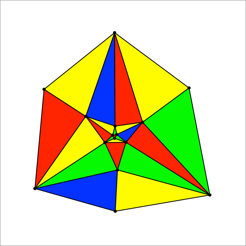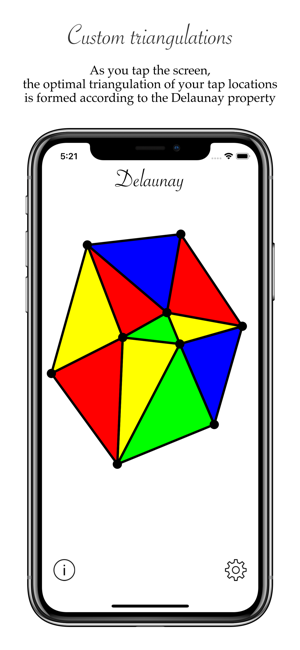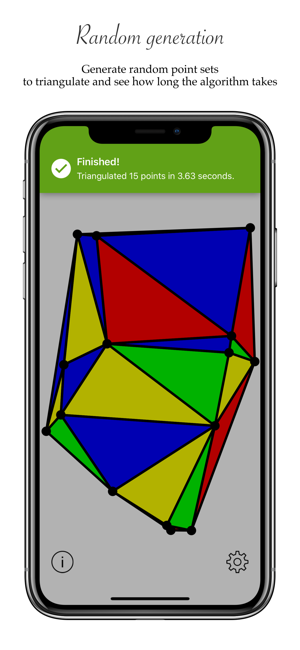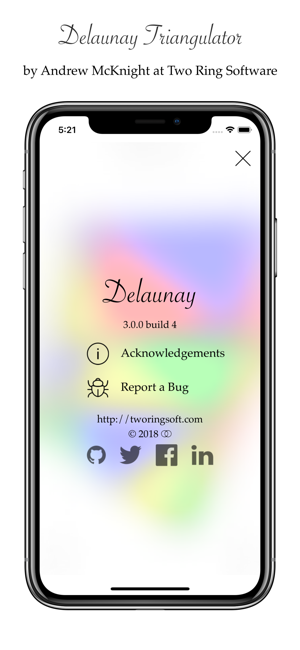## Screenshots

••••## Description

This app computes the Delaunay triangulation of a set of points in the plane, which you input by simply tapping the screen. You may also generate random point sets of different sizes to see how long the computation takes.

More on Delaunay triangulation:

For a given set of points on the plane, there exist many possible ways to triangulate them, that is, subdivide the plane into triangles where each point lies on a triangle vertex. Some triangulations produce very thin triangles with high aspect ratios. For a given triangulation, you may list all the angles from each triangle in a list, sorted by their magnitude from smallest to largest; call this the angle tuple for that triangulation. Now, if you take the set of angle tuples from all possible triangulations for the same set of points and sort them, then the largest lexicographic angle tuple is the one containing the largest minimal angle from the triangulation, meaning it is the triangulation that minimizes high aspect triangle slivers.

This is considered optimal since it restricts region boundaries to be the closest possible to the input points. Consider a map with a set of temperature readings from local weather stations. If you wanted to know what the temperature might be between stations, you must interpolate their readings. Triangulating is one way to do this, by setting the temperature of each triangle to the average of the readings from the stations at its vertices. Therefore, a triangulation with high aspect triangles may carry a reading to a place on the map far away from the weather station that recorded it. We'd use the Delaunay Triangulation in this case to optimize this interpolation.

The triangulation is constructed by inserting the points one by one. There are a couple things that can happen on each insertion. If it is the first or second point, no triangles are yet created. Now assume there is one triangle–three possibilities occur on inserting the next point:
1) it lies inside the triangle. In this case, it is split into three new triangles, all adjacent on that new point.
2) it lies outside the triangle. Then two edges are inserted adjacent to the two next closest vertices to create a neighboring triangle adjacent on the edge that joins those two vertices.
3) it lies on an edge of the triangle. In this case, the triangle(s) adjacent on the edge is(are) bisected with an edge joining the new point to the other vertex(vertices) in the triangle(s).
(A fourth possibility exists, if the point is identical to a point already in the triangulation; this is simply ignored.)

After constructing the new triangles, the new edges must be tested for the Delaunay property. This is done by drawing the circumcircle of a newly created triangle that contains the inserted point and the opposite edge, and testing whether the vertex in the neighboring triangle adjacent to that edge lies outside the circumcircle. If it lies on or outside the circumcircle, that edge is considered optimal. If it lies inside, then the edge is remove and a new edge is drawn between the new point and the opposite point tested for incircle orientation, creating two new, oppositely oriented triangles, whose other edges are subsequently tested. This edge legalization process propagates throughout the triangulation recursively until all edges pass the test.

Even more:
https://en.wikipedia.org/wiki/Delaunay_triangulation
https://www.researchgate.net/publication/221561628_Generalized_Delaunay_Mesh_Refinement_From_Scalar_to_Parallel
https://www.researchgate.net/publication/221561628_Generalized_Delaunay_Mesh_Refinement_From_Scalar_to_Parallel

## What’s New

Version 3.0.0

Rewritten in Swift and optimized for all the latest devices running iOS 12!

## Information

Seller
Two Ring Software LLC
Size
14.4 MB
Category
Entertainment
Compatibility

Requires iOS 11.0 or later. Compatible with iPhone, iPad, and iPod touch.

Languages

English

Age Rating
4+
Price
Free

## Supports

•